# RD Sharma Solutions for Class 6 Maths Chapter 14: Circles Exercise 14.1

The students can get a brief idea about the important concepts which are covered in this Chapter using the PDF of solutions. This exercise has problems based on the steps followed to draw a circle and methods of determining angles and diameters. The students can refer to the solutions while solving problems of RD Sharma textbook to gain expertise in the subject. To get a better conceptual knowledge of this Chapter, the students can download RD Sharma Solutions Class 6 Maths Chapter 14 Circles Exercise 14.1 PDF, which are provided here.

## RD Sharma Solutions for Class 6 Maths Chapter 14: Circles Exercise 14.1 Download PDF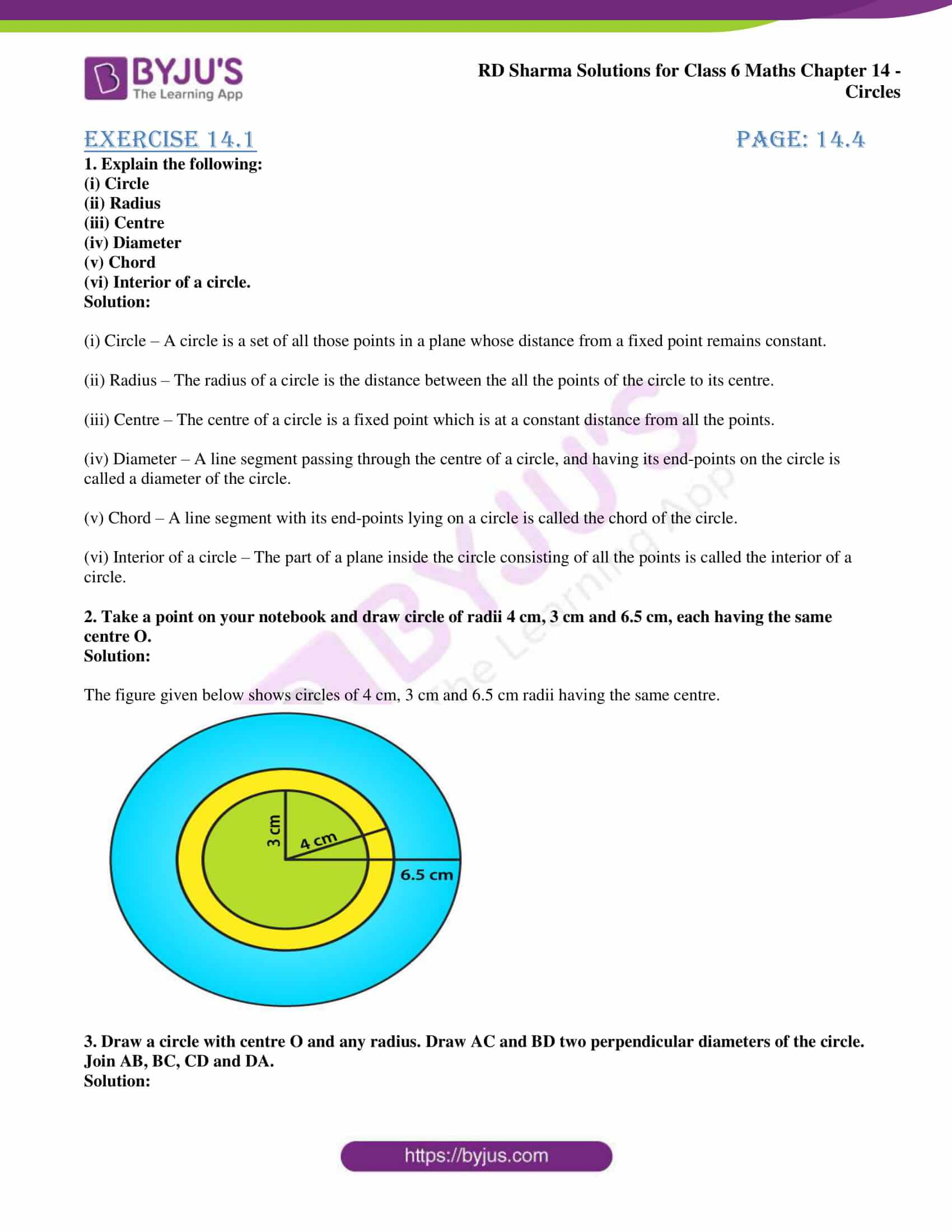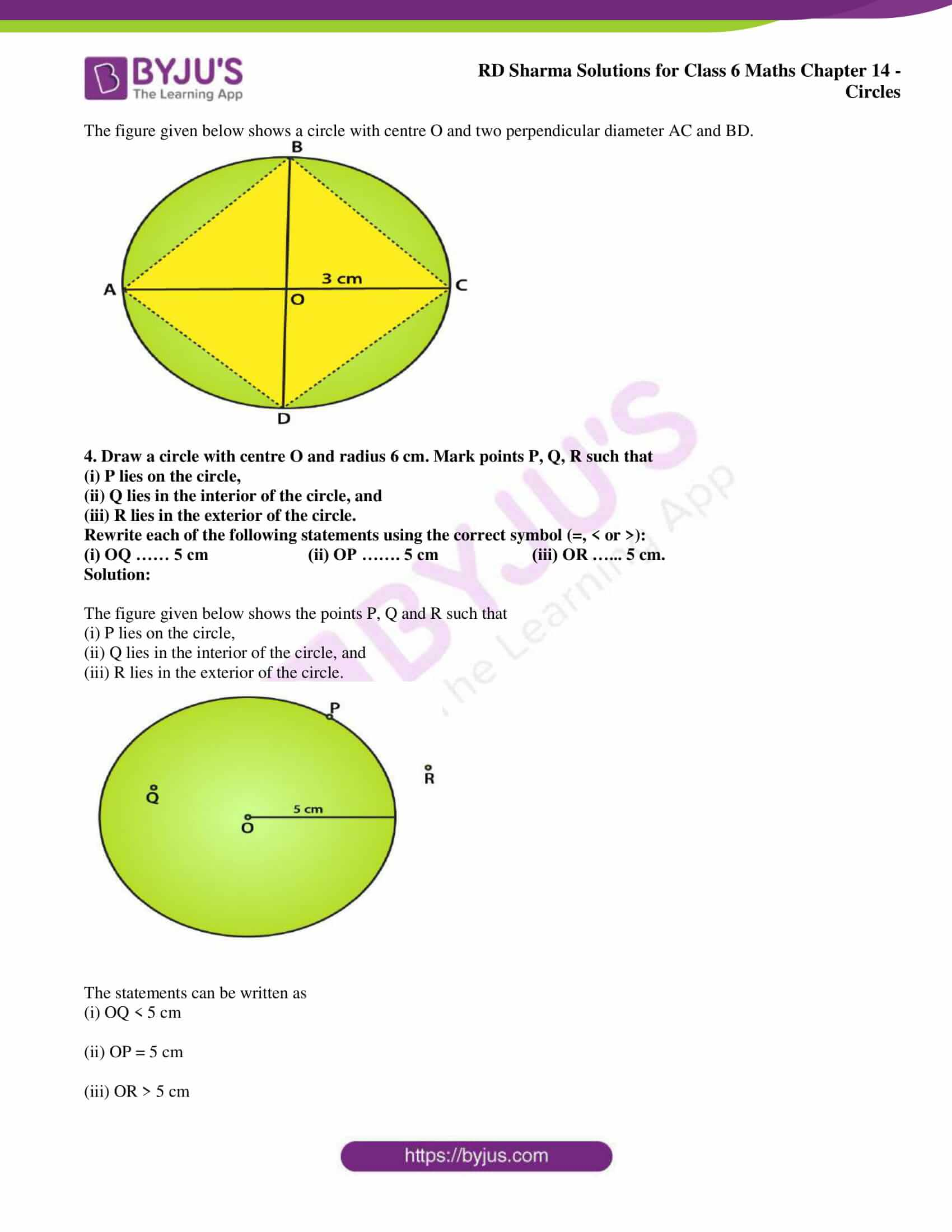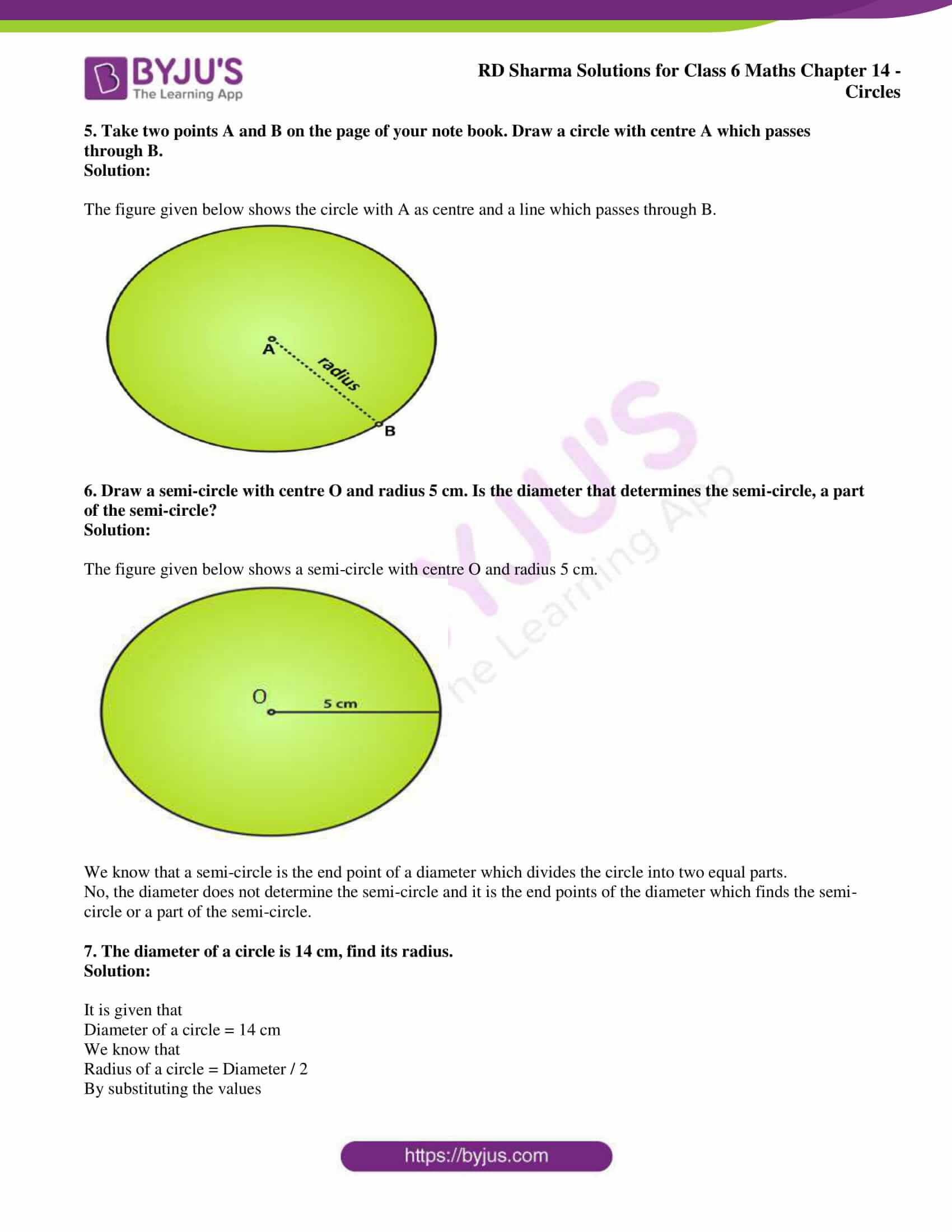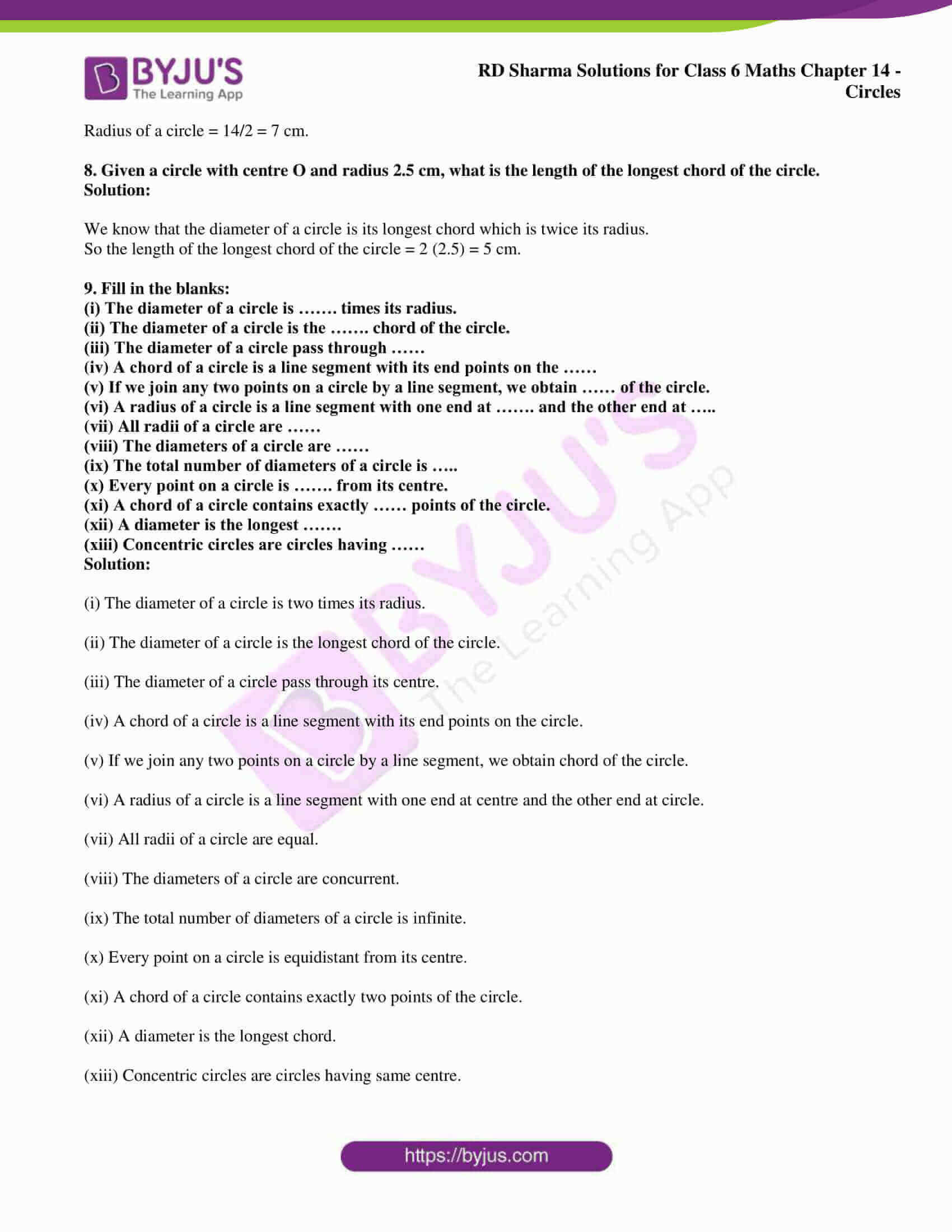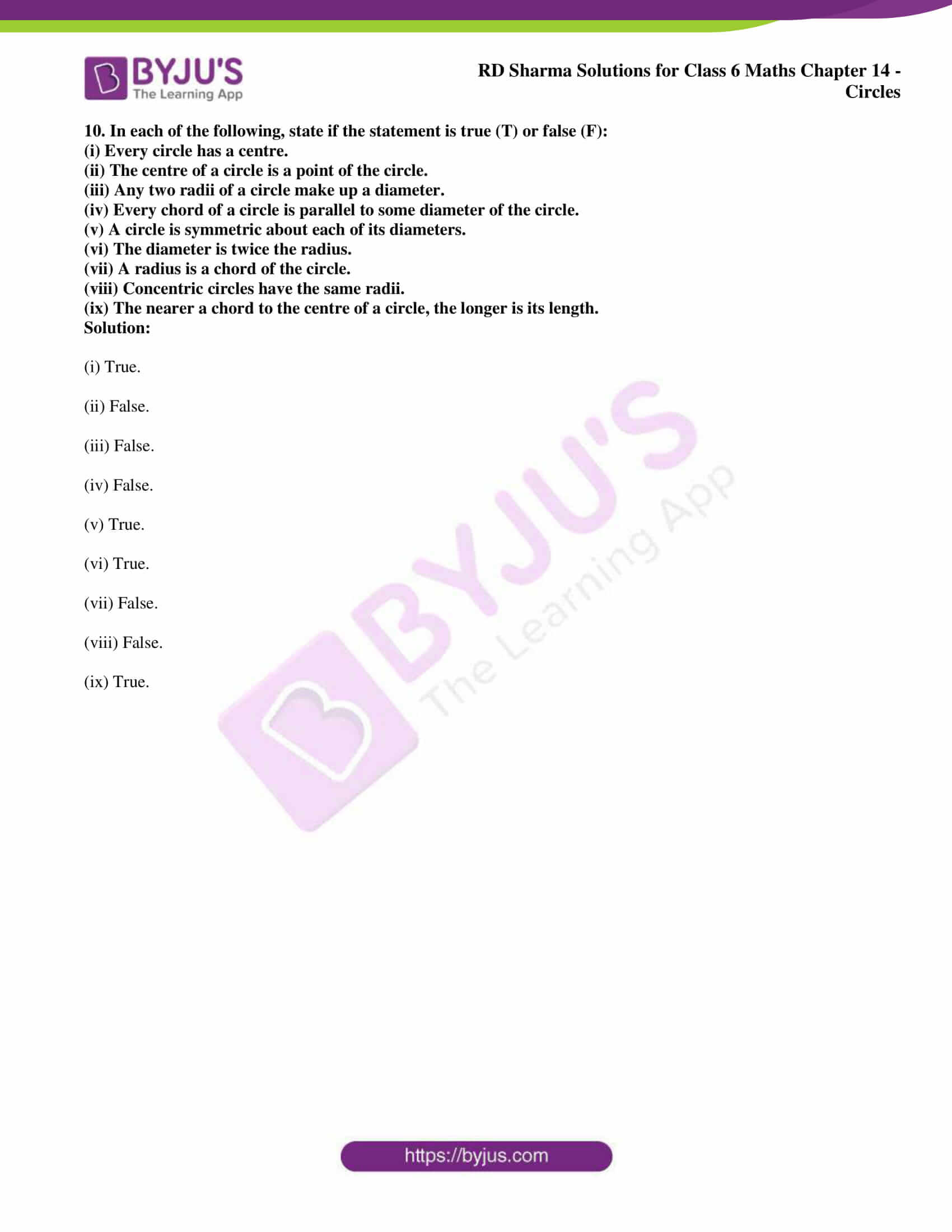### Exercise 14.1 page: 14.4

1. Explain the following:

(i) Circle

(iii) Centre

(iv) Diameter

(v) Chord

(vi) Interior of a circle.

Solution:

(i) Circle – A circle is a set of all those points in a plane whose distance from a fixed point remains constant.

(ii) Radius – The radius of a circle is the distance between the all the points of the circle to its centre.

(iii) Centre – The centre of a circle is a fixed point which is at a constant distance from all the points.

(iv) Diameter – A line segment passing through the centre of a circle, and having its end-points on the circle is called a diameter of the circle.

(v) Chord – A line segment with its end-points lying on a circle is called the chord of the circle.

(vi) Interior of a circle – The part of a plane inside the circle consisting of all the points is called the interior of a circle.

2. Take a point on your notebook and draw circle of radii 4 cm, 3 cm and 6.5 cm, each having the same centre O.

Solution:

The figure given below shows circles of 4 cm, 3 cm and 6.5 cm radii having the same centre.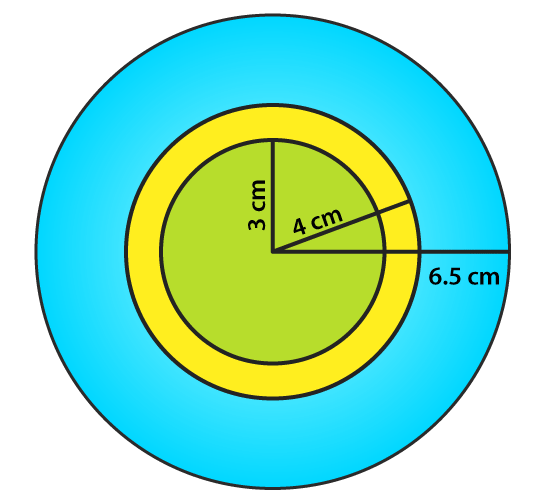3. Draw a circle with centre O and any radius. Draw AC and BD two perpendicular diameters of the circle. Join AB, BC, CD and DA.

Solution:

The figure given below shows a circle with centre O and two perpendicular diameter AC and BD.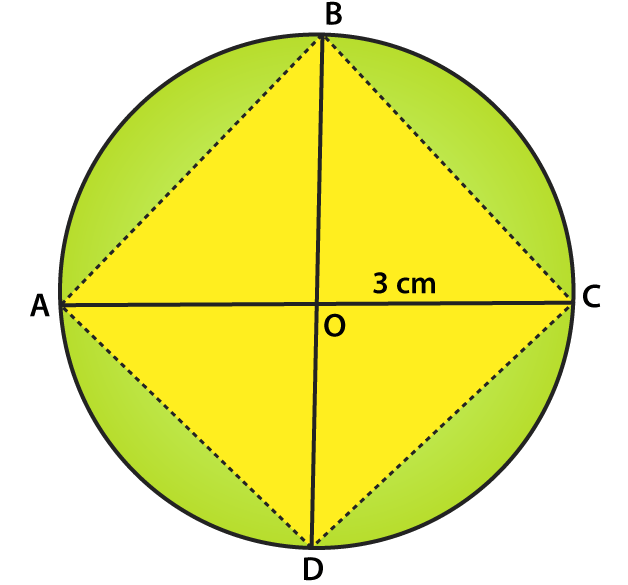4. Draw a circle with centre O and radius 6 cm. Mark points P, Q, R such that

(i) P lies on the circle,

(ii) Q lies in the interior of the circle, and

(iii) R lies in the exterior of the circle.

Rewrite each of the following statements using the correct symbol (=, < or >):

(i) OQ …… 5 cm (ii) OP ……. 5 cm (iii) OR …… 5 cm.

Solution:

The figure given below shows the points P, Q and R such that

(i) P lies on the circle,

(ii) Q lies in the interior of the circle, and

(iii) R lies in the exterior of the circle.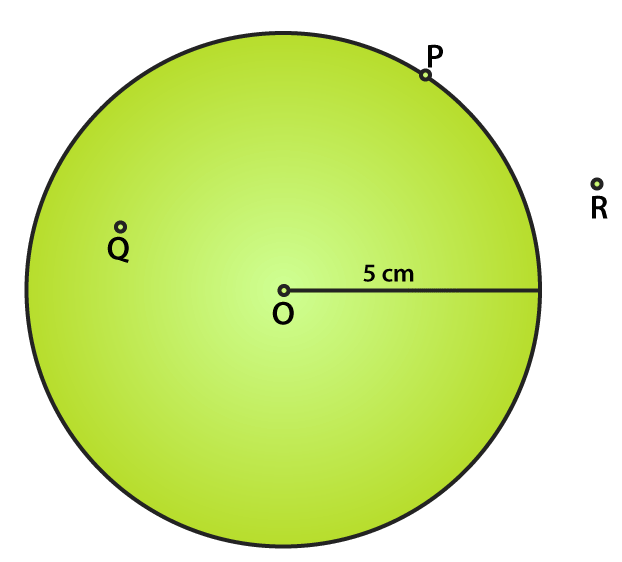The statements can be written as

(i) OQ < 5 cm

(ii) OP = 5 cm

(iii) OR > 5 cm

5. Take two points A and B on the page of your note book. Draw a circle with centre A which passes through B.

Solution:

The figure given below shows the circle with A as centre and a line which passes through B.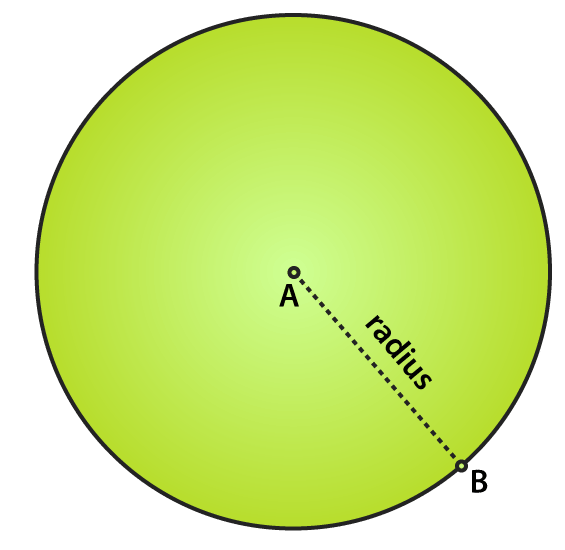6. Draw a semi-circle with centre O and radius 5 cm. Is the diameter that determines the semi-circle, a part of the semi-circle?

Solution:

The figure given below shows a semi-circle with centre O and radius 5 cm.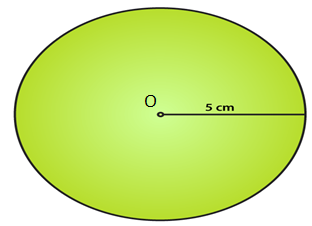We know that a semi-circle is the end point of a diameter which divides the circle into two equal parts.

No, the diameter does not determine the semi-circle and it is the end points of the diameter which finds the semi-circle or a part of the semi-circle.

7. The diameter of a circle is 14 cm, find its radius.

Solution:

It is given that

Diameter of a circle = 14 cm

We know that

Radius of a circle = Diameter / 2

By substituting the values

Radius of a circle = 14/2 = 7 cm.

8. Given a circle with centre O and radius 2.5 cm, what is the length of the longest chord of the circle.

Solution:

We know that the diameter of a circle is its longest chord which is twice its radius.

So the length of the longest chord of the circle = 2 (2.5) = 5 cm.

9. Fill in the blanks:

(i) The diameter of a circle is ……. times its radius.

(ii) The diameter of a circle is the ……. chord of the circle.

(iii) The diameter of a circle pass through ……

(iv) A chord of a circle is a line segment with its end points on the ……

(v) If we join any two points on a circle by a line segment, we obtain …… of the circle.

(vi) A radius of a circle is a line segment with one end at ……. and the other end at …..

(vii) All radii of a circle are ……

(viii) The diameters of a circle are ……

(ix) The total number of diameters of a circle is …..

(x) Every point on a circle is ……. from its centre.

(xi) A chord of a circle contains exactly …… points of the circle.

(xii) A diameter is the longest …….

(xiii) Concentric circles are circles having ……

Solution:

(i) The diameter of a circle is two times its radius.

(ii) The diameter of a circle is the longest chord of the circle.

(iii) The diameter of a circle pass through its centre.

(iv) A chord of a circle is a line segment with its end points on the circle.

(v) If we join any two points on a circle by a line segment, we obtain chord of the circle.

(vi) A radius of a circle is a line segment with one end at centre and the other end at circle.

(vii) All radii of a circle are equal.

(viii) The diameters of a circle are concurrent.

(ix) The total number of diameters of a circle is infinite.

(x) Every point on a circle is equidistant from its centre.

(xi) A chord of a circle contains exactly two points of the circle.

(xii) A diameter is the longest chord.

(xiii) Concentric circles are circles having same centre.

10. In each of the following, state if the statement is true (T) or false (F):

(i) Every circle has a centre.

(ii) The centre of a circle is a point of the circle.

(iii) Any two radii of a circle make up a diameter.

(iv) Every chord of a circle is parallel to some diameter of the circle.

(v) A circle is symmetric about each of its diameters.

(vi) The diameter is twice the radius.

(vii) A radius is a chord of the circle.

(viii) Concentric circles have the same radii.

(ix) The nearer a chord to the centre of a circle, the longer is its length.

Solution:

(i) True.

(ii) False.

(iii) False.

(iv) False.

(v) True.

(vi) True.

(vii) False.

(viii) False.

(ix) True.